## Wednesday, March 31, 2010

### Eric.P's Fraction Growing Post

Question 4 Part A:

1/4 ÷ 2 =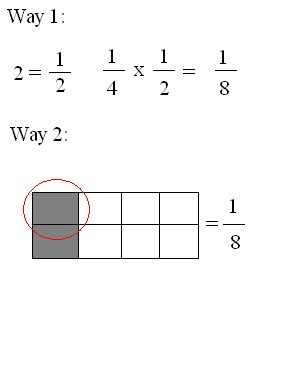Question 7:

A pitcher of orange juice is 2/3 full. If four students equally share the juice, what fraction of the full pitcher does each student get?

2 x 1 = 2

3 x 4 = 12

= 2/12

Each student gets 2/12 of the ful pitcher.

Part 2
Fraction x Fraction
3/4 x 7/8 =

Solution: Numerator x Numerator and Denominator x Denominator.
3 x 4 = 21
7 x 8 = 32

3/4 x 7/8 = 21/32

Mixed x Fraction
1 1/5 x 2/5 =

Solution: Convert mixed into improper. Then Numerator x Numerator and Denominator x Denominator.
(1x5)+1 = 6/5

(Whole x Denominator)+ Numerator = New Fraction

6/5 x 2/5 = 12/25

Mixed x Mixed
5 1/3 x 3 1/3 =

Solution: Covert Mixed fractions to improper. Then Numerator x Numerator and Denominator x Denominator. Then convert answer to mixed with remainder over denominator.

(5x3)+1 = 16/3
(3x3)+1 = 10/3

16/3 x 10/3 = 160/9
160 / 9 = 17 7/9

Part 3
Explain how to answer
1 1/2 ÷ 3/4 =
3/4 ÷ 1/2 =

If the denominator is not the same then you must find a common denominator.
Next you draw a box out of the denominator. Ex 1/4 you would draw a box divided in 4ths.
Now count up by the numerator until you can't count anymore. Ex 1/4 count up by 1.
With the left over make it a fraction with the numerator as a denominator.

1 1/2 ÷ 3/4 = 2
3/4 ÷ 1/2 = 1 1/2

Sorry theres no picture but everytime I put one something wierd happened.

### Abby's Fraction Growing post

What we have to do for our growing post:

Fraction Growing Post
Label it Fraction, Growing Post, User Name
a) Post your recorded quiz.
b) Using one question from questions 6 or 7 on page 202 of the textbook, explain two ways to multiply a fraction by a whole number. You should use pictures to help explain.
c) Using one question from questions 4 or 5 on page 208 of the textbook, explain how to divide a fraction by a whole number. You should use pictures.
d) Pick one word problem from questions 6-12 on page 208 of the textbook.

c) One question from #4 on page 208d) word problem from page 208 #7

Thanks for viewing my post. Sorry If I made any mistakes with my explanations. and sorry about my quiz too..
________________________________________________________________

Fraction Growing Post Part 2
________________________________

The second part of your fraction growing post is to show your understanding of multiplying fractions. Use your test to choose 3 questions.
1. fraction x fraction
2. fraction x mixed number
3. mixed number x mixed number
It is up to you how to show your understanding. You can type everything, create an image in paint or use the class ustream account. This is due by Monday April 12.

Yes there will be a part
3. DO NOT CREATE A NEW POST edit your old fraction post and add part 2 to it.
Be sure to label your post fractions and growingpost.

You also have to complete your e-portfolios and movie projects..... lots of homework. Have a great weekend.PART 3

Just click on the link to see.. It wont upload here and the embed thingy wont work..

## Friday, March 26, 2010

### Krystal's Growing Post

RECTANGULAR PRISMTOP;FRONT;SIDE

Area =LxW Area=
LxW Area=LxW
Area = 5x8 Area=8x8 Area=8x8
Area =40cm2 Area=64cm2 Area=64cm2

TSA= TOP + TOP + FRONT + FRONT + SIDE + SIDE
= 40+40+64+64+64+64
= 208 cm2TRIANGULAR PRISM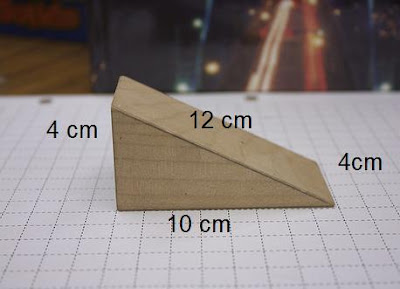a = b x h ÷ 2
a = 10 x 12 ÷ 2
a = 60cm²
a = l x w
a = 10 x 4
a = 40cm²

a= l x w
a = 10 x 4
a = 40cm²

a= l x w
a= 10x 12
a = 60cm²

= (triangle)+(triangle)+(rectangle)+(rectangle)+(rectangle)
= 60+60+60+40+40
= 260cm²

CylinderRadius:
r = d ÷ 2
r = 12 ÷ 2
r = 6cm²

Formula:
sa = 2 x (pi x r²) + (pi x d x h)
sa = 2 x 3.14 x 6 x 6 + 3.14 x 6 x 12
sa = 226.08+ 226.08

## Thursday, March 25, 2010

### Razel's Surface Area Growing Post

Rectangular prism

## Sunday, March 21, 2010

### Surface Area Growing PostRectangular Prism

Triangular PrismCylinder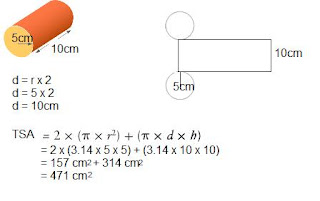Sorry i cant upload the video's but here the site where u can see it
http://www.ustream.tv/recorded/5208235
http://www.ustream.tv/recorded/5208364
http://www.ustream.tv/recorded/5208565

SORRY THE IT WAS LATE TOO
comment if i made some mistake

## Tuesday, March 16, 2010

### Surface Area growing post Richard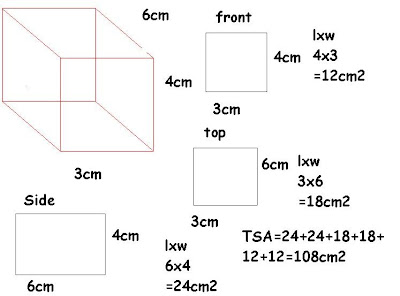Rectangular prismCylinder

formula 2x3.14xrxrx3.14xdxh 2x3.14x3x3x3.14x6x10=10648.40

Triangular PrismIf you want to find the surface area of a triangular prism you need to find the the area of the 2 triangles and the3 squares then add all the surface area of the 2 triangles and 3 squares then you should get the total surface area.

## Monday, March 8, 2010

### SURFACE AREA GROWING POST BY AMIEL

CYLINDER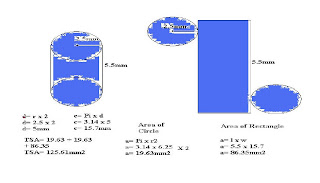TRIANGULAR PRISM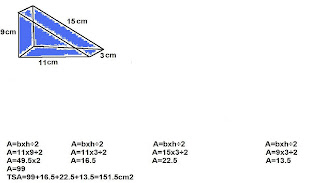RECTANGULAR PRISM### Kisha's Surface Area Growing Post

Rectangular Prism
Triangular Prism

Cylinder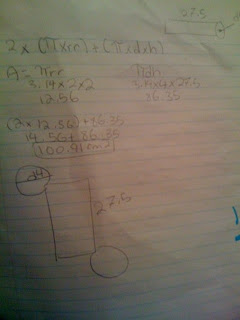Sorry I posted this late, its because Raquel and I worked so hard on the video, and it took like 3 hours to load onto youtube. So heres my post. And sorry if you can't really see my pictures. I did it in my notebook because it saves time from doing it in paint (:

## Sunday, March 7, 2010

### Surface Area Growing Post , JerickR873

Ok this is my growing post for math , i will be showing yo what a rectangular prism , triangular prism , and a cylinder looks like and nets , also how to solve for Total Surface Area (TSA)

1st the Rectangular Prismthe formula for the rectangular prism is:
l x w = a

one way to find TSA for a rectangle is to find the area of the side , front , and top then you just times those by two because of their opposite side

Top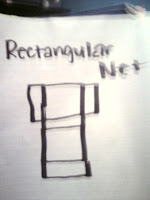l x w = a
20 x 2 =a
40x2=ax2
80=ax2

Side
l x w = a
20 x 2 = a
40 x 2 = ax2
80 = a x 2

Front

l x w = a
20 x 20 = a
400 x 2 = a x2
800 = a x2

TSA = 800+80+80
TSA= 960 cm22nd is the Triangular Prism

Formula
b x h = a
l x w = a
I like to find all sides just like the rectangular prism and add up all the sides

Front
b x h = a
12 x 12 = a
144 x 2=a
288 = a x2

Bottom
l x w = a
12 x 5 = a
60 = aBack
l x w = a
12 x 5 = a
60 = a

Top
l x w = a
1o x 5 = a
50 = a

TSA= 50 +60 + 60 + 288
TSA= 458 cm2

3rd the Cylinder

Formulas
d divided by 2 = r
pi R 2 = a
pi D = c
l x w = a

For cylinders , you need to find the area and circumference of a circle and times that by 2 , for the other end , and then for the rectangle its the circumference of the circle that is the width of the rectangle

pi D = C
3.14 x 15 = c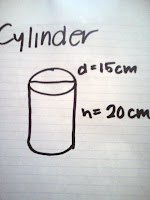47.1 = c

d/2 = r
15/2=r
7.5= r

pi R 2 = a
3.14 x 7.5(2) = a
3.14 x 56.26 = a
176.67 x 2 = a
353.34= a

l x w = a
20 x 47.1 = a
942=a942 + 353.54 =1295.34 cm2

Thanks YOU FOR READING MY POST

PLEASE COMMENT ON ANYTHING THAT I DID WRONG OR
THINGS I CAN DO BETTER